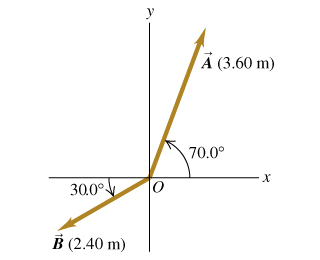# Problem: You may want to review (Pages 18 - 19). For related problemsolving tips and strategies, you may want to view a Video Tutor Solution of Using unit vectors.Write the vector A in the figure in terms of the unit vectors i and j .Write the vector B in the figure in terms of the unit vectors i and j .Use unit vectors to express the vector C, where = 3.00 - 4.00 .Find the magnitude of C.Find the direction of C.

⚠️Our tutors found the solution shown to be helpful for the problem you're searching for. We don't have the exact solution yet.

###### Problem Details

You may want to review (Pages 18 - 19).

For related problemsolving tips and strategies, you may want to view a Video Tutor Solution of Using unit vectors.

Write the vector A in the figurein terms of the unit vectors i and j .

Write the vector B in the figure in terms of the unit vectors i and j .

Use unit vectors to express the vector C, where = 3.00 - 4.00 .

Find the magnitude of C.

Find the direction of C.